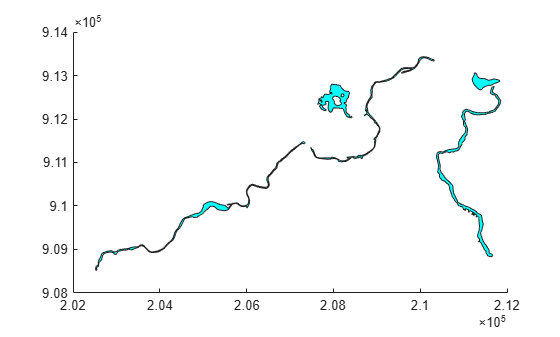# struct2geotable

Convert structure array to geospatial table

Since R2021b

## Syntax

``GT = struct2geotable(S)``
``GT = struct2geotable(S,coordinateSystemType,fnames)``
``GT = struct2geotable(S,Name=Value)``

## Description

example

````GT = struct2geotable(S)` converts the structure array `S` to a geospatial table `GT`. The function creates the `Shape` variable of `GT` by using the latitude-longitude or x-y coordinates in `S`. Each field of `S` is a variable of `GT`.```
````GT = struct2geotable(S,coordinateSystemType,fnames)` creates the `Shape` variable of `GT` by using the coordinate system type `coordinateSystemType` and structure array fields `fnames`.```
````GT = struct2geotable(S,Name=Value)` specifies options using name-value arguments. For example, specify that coordinates represent points, lines, or polygons by using the `GeometryType` name-value argument.```

## Examples

collapse all

Read a shapefile containing hydrographic data for Concord, MA, as a structure array. Import only the polygons with an area greater than 100 square kilometers. The x- and y-coordinates are in the `X` and `Y` fields of the structure.

```areafilterfcn = @(areafilter) (areafilter > 100000); S = shaperead("concord_hydro_area.shp", ... Selector={areafilterfcn,'AREA'});```

Get the coordinate reference system for the data.

```info = shapeinfo("concord_hydro_area.shp"); crs = info.CoordinateReferenceSystem;```

Convert the structure array to a geospatial table. The `struct2geotable` function detects the `X` and `Y` fields and uses them to create the `Shape` variable. Specify the coordinate reference system for the `Shape` variable.

```GT = struct2geotable(S, ... CoordinateReferenceSystem=crs);```

View the shape object in the second row of the table. Shape object properties contain information such as the number of holes.

`GT.Shape(2)`
```ans = mappolyshape with properties: NumRegions: 1 NumHoles: 3 Geometry: "polygon" CoordinateSystemType: "planar" ProjectedCRS: [1x1 projcrs] ```

Display the polygons.

`mapshow(GT,FaceColor="c")`Create a sample structure array containing the locations of cities. The `Lats` field contains latitudes, the `Lons` field contains longitudes, and the `Names` field contains names.

```lats = {35.7082,-22.8842,51.5074,39.9042,37.9838}; lons = {139.6401,-43.3882,-0.1278,116.4074,23.7275}; n = {'Tokyo','Rio de Janeiro','London','Beijing','Athens'}; S = cell2struct([lats;lons;n],{'Lats','Lons','Names'},1)```
```S=5×1 struct array with fields: Lats Lons Names ```

Convert the structure array to a geospatial table. The `struct2geotable` function does not automatically detect the field names `Lats` and `Lons`. Specify the coordinate system type as `"geographic"` and the field names containing the coordinates.

`GT = struct2geotable(S,"geographic",["Lats" "Lons"])`
```GT=5×4 table Shape Lats Lons Names _______________________ _______ _______ __________________ (35.7082°N, 139.6401°E) 35.708 139.64 {'Tokyo' } (22.8842°S, 43.3882°W) -22.884 -43.388 {'Rio de Janeiro'} (51.5074°N, 0.1278°W) 51.507 -0.1278 {'London' } (39.9042°N, 116.4074°E) 39.904 116.41 {'Beijing' } (37.9838°N, 23.7275°E) 37.984 23.727 {'Athens' } ```

View the `Shape` variable of the geospatial table.

`GT.Shape`
```ans = 5x1 geopointshape array with properties: NumPoints: [5x1 double] Latitude: [5x1 double] Longitude: [5x1 double] Geometry: "point" CoordinateSystemType: "geographic" GeographicCRS: [] ```

## Input Arguments

collapse all

Input structure array.

The `struct2geotable` function detects the coordinates when the structure array fields have these names, ignoring case.

• Latitude coordinates — `Latitude` or `Lat`

• Longitude coordinates — `Longitude`, `Lon`, or `Long`

• x-coordinates — `X`

• y-coordinates — `Y`

If you specify an input structure array with a field called `Shape`, then the `struct2geotable` function overwrites it.

Data Types: `struct`

Coordinate system type, specified as one of these options:

• `"planar"` — Coordinates are in a planar coordinate system.

• `"geographic"` — Coordinates are in a geographic coordinate system.

Data Types: `string`

Names of the structure array fields used to create the `Shape` variable of the geospatial table, specified as a two-element string vector, such as `["Lats" "Lons"]`. The fields contain latitude-longitude or x-y coordinates.

Data Types: `string`

### Name-Value Arguments

Specify optional pairs of arguments as `Name1=Value1,...,NameN=ValueN`, where `Name` is the argument name and `Value` is the corresponding value. Name-value arguments must appear after other arguments, but the order of the pairs does not matter.

Example: `struct2geotable(S,CoordinateReferenceSystem=geocrs(4326))` specifies the geographic CRS as the World Geodetic System of 1984, which has EPSG code `4326`.

Coordinate reference system (CRS) to use when creating the `Shape` variable of the geospatial table, specified as a `projcrs` or `geocrs` object.

Specify a `projcrs` object when `coordinateSystemType` is `"planar"` and specify a `geocrs` object when `coordinateSystemType` is `"geographic"`.

Geometry type of the coordinate fields, specified as one of these options:

• `"point"` — The coordinate fields represent points.

• `"line"` — The coordinate fields represent lines.

• `"polygon"` — The coordinate fields represent polygons.

When you specify the geometry type as `"polygon"`, the `struct2geotable` function assumes that the coordinates define polygons with a valid topology. A polygon has a valid topology when:

• Region interiors are to the right as you trace boundaries from vertex to vertex.

• The boundaries have no self-intersections.

In general, the outer boundaries of polygons with a valid topology have vertices in a clockwise order and the interior holes have vertices in a counterclockwise order.

The `struct2geotable` function detects the geometry type when the input structure array has a `Geometry` field with a value of `'Point'`, `'Multipoint'`, `'Line'`, or `'Polygon'`.

## Output Arguments

collapse all

Output geospatial table. A geospatial table is a `table` or `timetable` object with a `Shape` variable that contains `geopointshape`, `geolineshape`, `geopolyshape`, `mappointshape`, `maplineshape`, or `mappolyshape` objects.

For an input structure array with M elements and N fields, the size of `GT` is M-by-(N + 1).

## Tips

• When the input structure has latitude-longitude fields and x-y fields, the `struct2geotable` function creates the geospatial table using the latitude-longitude fields. Create the geospatial table using the x-y fields by specifying the `coordinateSystemType` and `fnames` arguments.

• When you know that a shapefile contains latitude and longitude coordinates and read the shapefile using the `shaperead` function, you must specify the `UseGeoCoords` argument as `true`. Otherwise, the structure array contains `X` and `Y` fields instead of `Lat` and `Lon` fields. As a result, the `struct2geotable` function creates the `Shape` variable of the geospatial table using planar shape objects such as `mappointshape` instead of geographic shape objects such as `geopointshape`.

## Version History

Introduced in R2021b